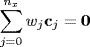Chapter 10

The Sigma Point Class: The Spherical Simplex Kalman Filter

For the UKF, with the exception of the integration point at the origin, the vector integration points used for the moment integrations (9.9)(9.11) are symmetrical about 0, equidistant from the origin, and fall on the axes of an nx-dimensional Cartesian coordinate system. For the spherical simplex Kalman filter, we replace the requirement for symmetry about the origin with a requirement that all vector points be equidistant from the origin and from each other, thus lying at the vertices of an nx-dimensional simplex (see Figure 2.4). This integration rule was first proposed by Julier [10Julier].

Let the integration (sigma) points be redefined asAll of these simplex vector points cj must satisfy the moment equations (9.9)(9.11), repeated here for clarity

(10.1)(10.2)(10.3)Get Bayesian Estimation and Tracking: A Practical Guide now with the O’Reilly learning platform.

O’Reilly members experience live online training, plus books, videos, and digital content from nearly 200 publishers.